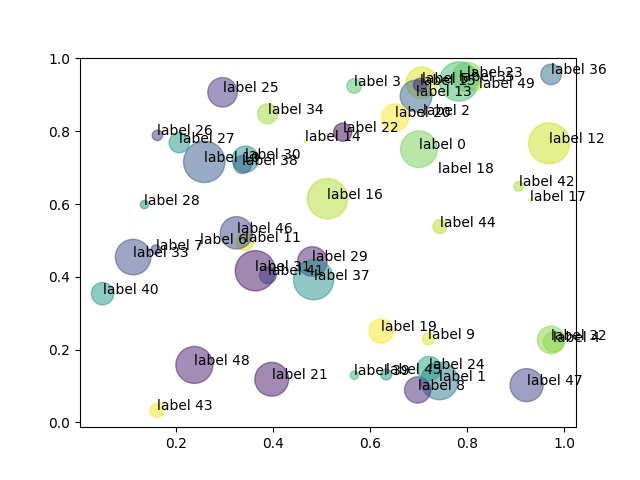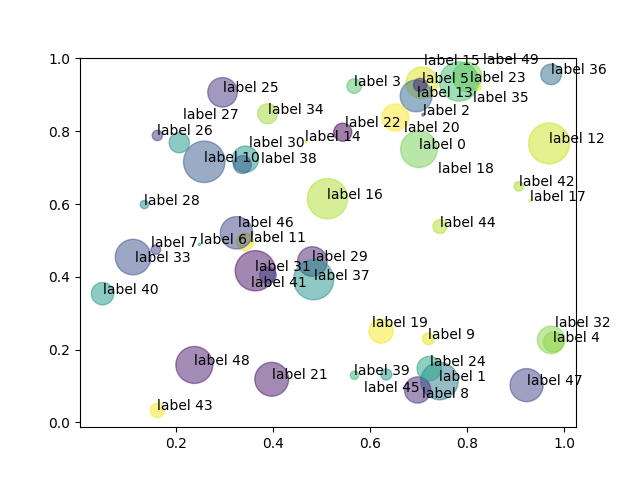No project description provided

# overlab

This is a function to prevent overlapping when annotating a plot in matplotlib. The algorithm works by starting from the coordinate to annotate and slowly moving the label in a spiral centered in the starting point progressively distancing from it until find a suitable place that is not overlapping any of the other labels.

The function is built on top of ax.annotate and takes the same **kwargs. The difference is that differently from ax.annotate that takes as argument single desired annotation and coordinates, overlab takes as arguments 3 iterables (x, y, and the list of labels) and the target ax).

## Installation

The package can be installed with

pip install overlab



## Example

#### No overlab


import numpy as np
import matplotlib.pyplot as plt

# Fixing random state for reproducibility
np.random.seed(19680801)

N = 50
x = np.random.rand(N)
y = np.random.rand(N)
colors = np.random.rand(N)
area = (30 * np.random.rand(N))**2  # 0 to 15 point radii
annotations=[f'label {str(n)}' for n in range(N)]

fig, ax = plt.subplots()
ax.scatter(x, y, s=area, c=colors, alpha=0.5)
for cordx, cordy, label in zip(x, y, annotations):
ax.annotate(label,(cordx,cordy))

plt.show()#### overlab

import numpy as np
import matplotlib.pyplot as plt
import overlab as ol

# Fixing random state for reproducibility
np.random.seed(19680801)

N = 50
x = np.random.rand(N)
y = np.random.rand(N)
colors = np.random.rand(N)
area = (30 * np.random.rand(N))**2  # 0 to 15 point radii
annotations=[f'label {str(n)}' for n in range(N)]

fig, ax = plt.subplots()
ax.scatter(x, y, s=area, c=colors, alpha=0.5)
ol.annoatate(x,y,annotations,ax=ax)

plt.show()## Project details

This version0.2.50.2.40.2.30.2.20.2.10.2.00.1.90.1.80.1.70.1.60.1.50.1.40.1.30.1.20.1.10.1.0

Uploaded py3